Scan QR code or get instant email to install app

Question:

# 1212What is the value of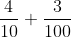?

A 1212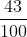explanation

1212The expression can be rewritten as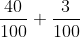, which is equal to.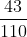is incorrect because it results from combining the numerators to create a two-digit number and adding the denominators.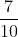is incorrect because it results from adding the numerators and using the denominator of the first number in the sum.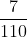is incorrect because it results from adding the numerators and denominators separately.